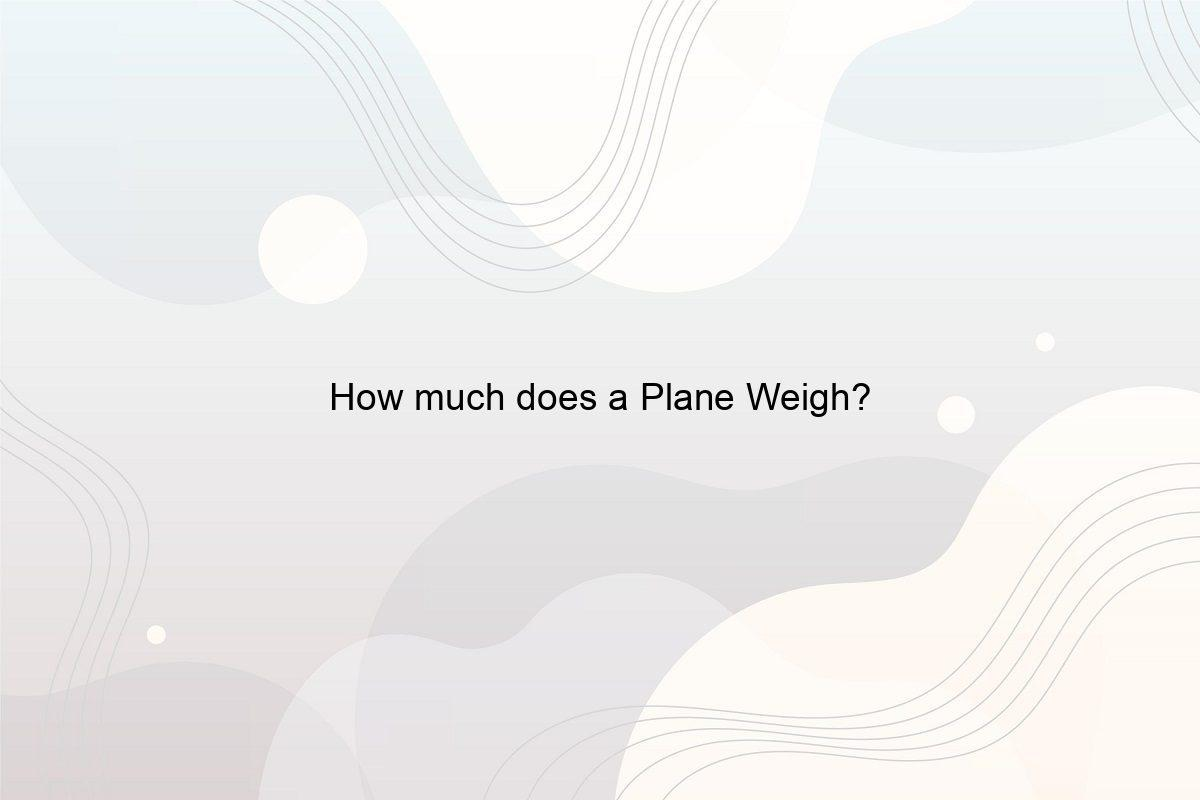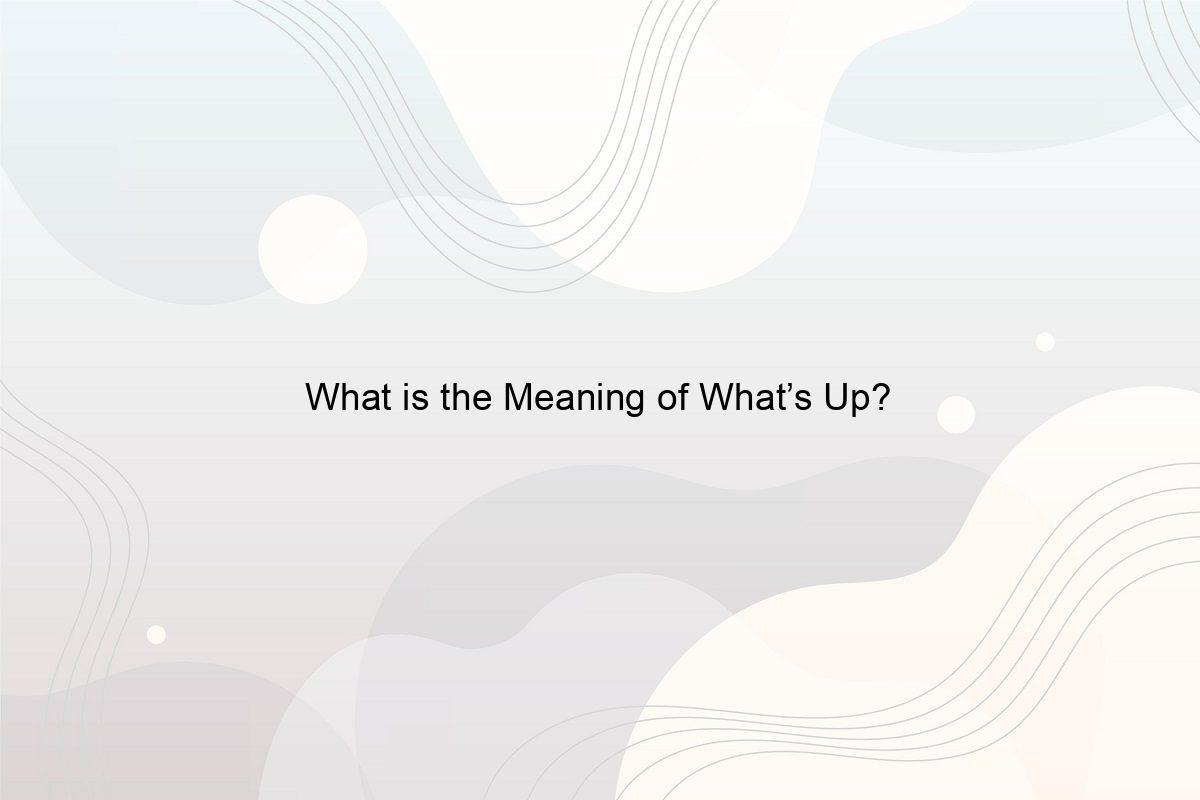﻿ How many Gallons of Water are in the Entire Ocean? - Speeli

# How many Gallons of Water are in the Entire Ocean?

How many Gallons does Each Ocean have? How much of the Ocean is just Water, and How much is Ice? Can Humans use all the Water? What is the Estimated Distribution of Water in Different Resources on the Earth?

Water is the source of life and its presence makes Earth the only planet that supports life. From the space view of our Earth, it is mostly water which is why it came to be known as Blue Planet. You already know that about 70% of the surface of the Earth is covered with water, but do you know how much water is it? How many gallons of water are in the entire ocean? If not, need not worry, along with these questions we’ll discuss how much of the ocean is just water and how many gallons of water are in the Atlantic.

### 1. How many Oceans are there?

Up till now, you have learned that there are 4 oceans namely,

• Atlantic Ocean
• Arctic Ocean
• Indian Ocean
• Pacific Ocean

But according to the United States Board of Geographic Names, the Southern Ocean is the fifth ocean. It is also known as the name Antarctic Ocean. Check out Which is the Largest and Smallest Oceans?

### 2. How many Gallons of Water are in the Entire Ocean?

We have to take all the water that is present in oceans along with the water that is evaporated. Considering their depth which is almost 12,100 feet (3,688 meters) we have 98% of the water on the Earth is in the oceans. Talking about all the oceans, there are about 326 million trillion gallons of water in them. Must read what is the main source of water?

### 3. How many Gallons of Water is in the Pacific Ocean?

Photo by Nate Kadlac on Unsplash

It is the largest ocean that stretches between America and Eurasia. It is divided into two parts by the equator, namely the South Pacific and North Pacific. The Pacific Ocean covers an area of about 165,250,000 kilometers square (63,800,000 square miles) that makes up about 20% of the surface of the Earth. The amount of water in the Pacific Ocean is 352 quintillion gallons (13,590,000,000,000,000,000 liters) of water. This value is almost close to how many gallons of water are in the entire ocean. (See What is the Difference Between a Sea and Ocean?)

### 4. How many Gallons of water are in the Atlantic Ocean?

The surface area covered by the Atlantic Ocean is about 106.5 million kilometers square (41,100,000 square miles), which is about 20% of the surface of the Earth. According to this, about one-fifth of the surface of the Earth is under the water of the Atlantic Ocean. The amount of water is 310,410,900 cubic kilometers of water. Here,

1 cubic kilometer of water = 264.17 billion gallons

Therefore, as per the calculation, the Atlantic Ocean has 82 billion gallons of water. (See How many ml in a Gallon?)

### 5. What is the Amount of Water in the Indian Ocean?

After reading how many gallons of water are in the entire ocean, it will be interesting to know about the water content in individual oceans. The Indian Ocean is the 3rd largest ocean in the world, and it stretches from South Asia to West Africa. The Indian Ocean covers about 70,560,000 kilometers square (27,240,000 square miles) surface area, which is almost 19.8% of the surface of the Earth. In terms of gallons, the Indian Ocean has 17,543,940,979,332,434 gallons of water. (See Large Body of Seawater is called?)

### 6. How much Water is in the Southern Ocean?

Photo by Henrique Setim on Unsplash

It is also known as the Antarctic Ocean that encircles Antarctica towards the south of 60°S latitude. It is the 2nd largest ocean in the world and covers an area of about 20,327,000 kilometers square. The Antarctic Ocean has 17,226,000 cubic miles (71,800,000 cubic kilometers) of water. (See How many Ounces in a Half Gallon?)

### 7. How much Water does the Arctic Ocean Have?

It is the smallest and shallowest ocean in the world that is around the North Pole. It covers a surface area of about 14,060,000 kilometers square (5,430,000 square miles) and about 1.3% of the surface of the Earth. The Arctic Ocean has a volume of 18.07 × 10ˆ6 kilometers cube, which is about 3.921 Quintilian gallons of water. (See What is the Very Bottom of the Ocean called?)

### 8. How much of the Ocean is just Water?

Water in the ocean is just water but the water that is evaporated from the oceans and floats in the atmosphere as water vapor is also water. Moreover, the water received by the oceans through precipitation is also water. After getting to know about how many gallons of water are in the entire ocean, take a look at how much is just water.

Taking into consideration all the water which makes it about 332,500,000 cubic miles or 1,386,000,000 cubic kilometers and about 860 miles (about 1,385 kilometers) in diameter. (See How to Describe Water?)

### 9. How much is Ice Water?

Photo by Jeremy Bishop on Unsplash

When you talk about water on the Earth and the ocean, you should take into consideration all the ice caps and glaciers present in them. The oceans near the poles are not just water but frozen layers of ice and snow. About 1.6% of the water of the Earth is in the form of polar ice caps and glaciers. (See Why Ice floats on Water?)

### 10. Is All Water usable by Humans?

No, out of 98% of water present on the Earth, only 96% of water is saline water of the oceans, which makes it unusable for drinking. The only water that is fit for consumption is fresh water that is received from rain, river, lakes, groundwater, wells, etc. The amount of freshwater present underground is more than the water present on the surface. (See What are the Uses of Water in our Daily Life?)

### 11. What is the Total Estimate of Water Distribution on the Earth?

Photo by NASA on Unsplash

Here is a list of all the places where water is present. I have mentioned the amount of water in these resources which will be useful while finding how many gallons of water are in the entire ocean.

• Atmosphere: 3,095 cubic meters (12,900 cubic kilometers) with freshwater content of about 0.04% making the total percentage about 0.001%.
• Bays, Oceans, and Seas: 321,000,000 cubic meters (1,338,000,000 cubic kilometers) and total percentage is 96.54.
• Biological Water: 269 cubic meters (1,120 cubic kilometers) with 0.003 % freshwater and a total percentage of 0.0001.
• Ground Ice and Permafrost: 71,970 cubic meters (300,000 cubic kilometers) and total percentage 0.022 with 0.86 % of freshwater.
• Groundwater: 5,614,000 cubic meters (23,400,000 cubic kilometers) with a total percentage of 1.69.
• Groundwater Fresh: 2,526,000 cubic meters (10,530,000 cubic kilometers) with a total percentage of 0.67 and 30.1% of freshwater content.
• Groundwater Saline: 3,088,000 cubic meters (12,870,000 cubic kilometers) making a total percentage of 0.93%.
• Lakes Total: 42,320 cubic meters (176,400 cubic kilometers) making a total of 0.013%.
• Lakes Fresh: 21,830 cubic meters (91,000 cubic kilometers) with 0.26% freshwater content which makes a total of 0.007%.
• Lakes Saline: 20,490 cubic meters (85,400 cubic kilometers) without any freshwater content making a total of 0.006%.
• Permanent snow, ice caps, and glaciers: 5,773,000 cubic meters (24,064,000 cubic kilometers) with 68.7% of freshwater making a total of 1.74%.
• Rivers: 509 cubic meters (2,120 cubic kilometers) with 0.006% of freshwater makes it 0.0002%.
• Soil Moisture: 3,959 cubic meters (16,500 cubic kilometers) with 0.05% freshwater making it a total of about 0.001%.
• Swamp Water: 2,752 cubic meters (11,470 cubic kilometers) with freshwater content up to 0.03% making a total of 0.0008%.

The answer to how many gallons of water are in the entire ocean is billions and millions of gallons. How much of the ocean is just water? Who could have imagined that it would be 96-98%? Did you find how many gallons of water are in the Atlantic and other major oceans of the world? (See What is the Largest Man-made Lake in USA?)

##### Related Posts## How much does a Plane Weigh?

How much does a 747 Airplane Weigh? How Heavy is the Heaviest Plane? What does One Gallon of Jet Fuel Weigh? How Fast do Planes fly in mph## What is POI in Trading?

What is meant by Poi in Banking, Trading and Food? What is used in making this Dish? How to eat Poi? Where to get Poi?